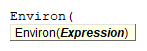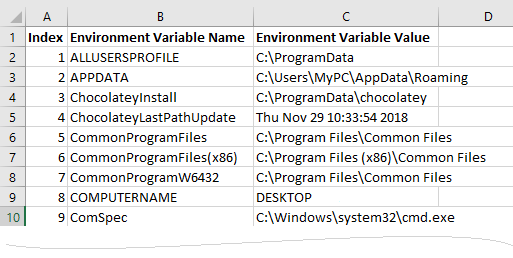# VBA Environ Functions

## Environ Description

Returns the value of an operating system environment variable.

## Simple Environ Examples

``````Sub Environ_Example()
End Sub
``````

This will returns the current user name logged in.

``````Sub Environ_Example()
MsgBox Environ("ComputerName")
End Sub
``````

This will returns the computer name.

## Environ Syntax

In the VBA Editor, you can type  “Environ(” to see the syntax for the Environ Function:The Environ function contains an argument:

Expression:  Name of an environment variable (string) or Numeric expression(1 to 255) corresponding to the numeric order of the environment string in the environment-string table.

## Examples of Excel VBA Environ Function

``MsgBox Environ(1)``

Result: “ALLUSERSPROFILE=C:\ProgramData”

``MsgBox Environ(0)``

Result: Run-time Error ‘5’

``MsgBox Environ(256)``

Result: Run-time Error ‘5’

``MsgBox Environ("AllUsersProfile")``

Result: “C:\ProgramData”

To see all environment variables that is defined on your computer, you can use the following code.

``````Sub ListAllEnvironVariables()
Dim strEnviron As String
Dim VarSplit As Variant
Dim i As Integer, nRow As Integer

nRow = 1
Range("A1").Value = "Index"
Range("B1").Value = "Environment Variable Name"
Range("C1").Value = "Environment Variable Value"
Range("A:C").Columns.AutoFit
Range("A1:C1").Font.Bold = True
nRow = 2

For i = 1 To 255
strEnviron = Environ(i)
If strEnviron <> "" Then
VarSplit = Split(strEnviron, "=")
Range("A" & nRow).Value = i
Range("B" & nRow).Value = VarSplit(0)
Range("C" & nRow).Value = VarSplit(1)
nRow = nRow + 1
End If
Next
End Sub``````

Then, you can see all environment variables as following.The result may vary by system. Because there are some custom environment variables besides the default environment variables.

For any string that doesn’t exist on the list of environment variables, it will return empty string(“”).

``MsgBox Environ("MyEnviron")``

Result: “”

Stop searching for VBA code online. Learn more about AutoMacro - A VBA Code Builder that allows beginners to code procedures from scratch with minimal coding knowledge and with many time-saving features for all users!